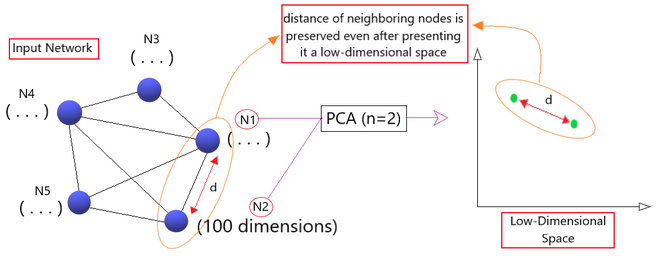# Node2Vec Algorithm

• Last Updated : 13 Dec, 2021

Prerequisite: Word2Vec

Word Embedding: It is a language modeling technique used for mapping words to vectors of real numbers. It represents words or phrases in vector space with several dimensions. Word embeddings can be generated using various methods like neural networks, co-occurrence matrix, probabilistic models, etc.

Word2Vec: It consists of models for generating word embedding.

Node2Vec: A node embedding algorithm that computes a vector representation of a node based on random walks in the graph. The neighborhood nodes of the graph is also sampled through deep random walks. This algorithm performs a biased random walk procedure in order to efficiently explore diverse neighborhoods.

It is based on a similar principle as Word2Vec but instead of word embeddings, we create node embeddings here.

Intuition:

Node2Vec framework is based on the principle of learning continuous feature representation for nodes in the graph and preserving the knowledge gained from the neighboring 100 nodes.  Lets us understand how the algorithm works.  Say we have a graph having a few interconnected nodes creating a network. So, Node2Vec algorithm learns a dense representation (say 100 dimensions/features) of every node in the network.

The algorithm suggests that if we plot these 100 dimensions of each node in a 2 dimensional graph by applying PCA, the distance of the two nodes in that low-dimensional graph would be same as their actual distance in the given network.

In this way, the framework maximizes the likelihood of preserving neighboring nodes even if you represent them in a low-dimensional space. (As shown in Fig 1)Fig1 : Intuition

Real-Life Example:

Let us take an example of textual data given to us to understand its working in detail. The Node2Vec framework suggests that random walks through the series of nodes in the graph can be treated as sentences. Each node in this graph is treated like a unique, individual word and each random walk through the network is treated as a sentence.

By creating and using a “continuous bag of words model” on which the Node2Vec framework learns, it can predict the next possible set of words by searching the neighbours of the given word. This is the power of Node2Vec algorithm.

Node2Vec Algorithm:

```node2vecWalk (Graph G' = (V, E, π), Start node u, Length l):

Initialize walk to [u]
for (walk_iter = 1 to l):
curr = walk[−1]
Vcurr = GetNeighbors(curr, G')
s = AliasSample(Vcurr, π)
Append s to walk
return walk```
```Learn_Features (Graph G = (V, E, W)):

Dimensions -> d
Walks per node -> r
Walk length -> l
Context size -> k
Return -> p
In-out -> q

π = PreprocessModifiedWeights    (G, p, q)
G' = (V, E, π)

Initialize walks = 0

for (iter = 1 to r):
for (all nodes u ∈ V):
walk =     node2vecWalk(G', u, l)
Append walk to walks